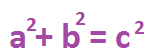# What is a Theorem? - Definition & Examples

An error occurred trying to load this video.

Try refreshing the page, or contact customer support.

Coming up next: What is Subtraction with Regrouping?

### You're on a roll. Keep up the good work!

Replay
Your next lesson will play in 10 seconds
• 0:00 Theorem Defined
• 0:38 The Pythagorean Theorem
• 1:24 Pythagorean Theorem Example
• 2:06 Triangle Sum Theorem
• 2:31 Triangle Sum Theorem Example
• 2:56 Lesson Summary
Save Save

Want to watch this again later?

Timeline
Autoplay
Autoplay
Speed Speed

#### Recommended Lessons and Courses for You

Lesson Transcript
Instructor: Allison Petrovic

Allison has experience teaching high school and college mathematics and has a master's degree in mathematics education.

In this lesson, will learn the definition of a theorem. We will also go over the Pythagorean theorem and the triangle sum theorem. An example of each theorem in action will also be provided.

## Theorem Defined

Have you ever heard of the word 'theorem'? Maybe you've heard of the Pythagorean theorem or the Triangle Sum theorem. The word 'theorem' is quite common in mathematics. As you continue to middle school, and even into high school, you'll learn many new theorems in your math classes.

So what is a theorem? Put simply, a theorem is a math rule that has a proof that goes along with it. In other words, it's a statement that has become a rule because it's been proven to be true. This definition will make more sense as we look over two popular theorems in mathematics. Let's get started!

## The Pythagorean Theorem

The Pythagorean theorem might be one of the most well known theorems in mathematics. This theorem explains that if you add together the squares of the two legs of a right triangle, you'll get the square of the hypotenuse. The hypotenuse is the side of a right triangle that is opposite from the right angle. So if we have a right triangle, we can label one of the shorter sides as a, and the other shorter side as b. We also can label the hypotenuse or the longest side as c. The formula for the Pythagorean theorem is then:The Pythagorean theorem will only work for triangles with a right angle. Let's not forget that every theorem has a proof. While there are many interesting proofs for this theorem, in this lesson we're just looking at the main idea.

To unlock this lesson you must be a Study.com Member.

### Register to view this lesson

Are you a student or a teacher?

#### See for yourself why 30 million people use Study.com

##### Become a Study.com member and start learning now.
Back
What teachers are saying about Study.com

### Earning College Credit

Did you know… We have over 200 college courses that prepare you to earn credit by exam that is accepted by over 1,500 colleges and universities. You can test out of the first two years of college and save thousands off your degree. Anyone can earn credit-by-exam regardless of age or education level.